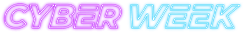#### Save big!

All courses under \$5 - for a limited time!

Code has been added to clipboard!

# Essentials of JavaScript Functions: How to Add Functions in JavaScript

Published Aug 8, 2017
Updated Oct 15, 2019

For a program to work, the computer needs some specific instructions on what, how, when, and where tasks need to be done. In JavaScript, we use functions to define these steps for the browser. In this tutorial, you'll learn about JavaScript functions, what they are, and how they are written.

We will introduce you to JavaScript function arguments which are passed to functions as values, and JS function parameters, which refer to the names included in the function. You'll learn about correct JavaScript function syntax as well.

## JavaScript Function: Main Tips

• When something calls (invokes) a function, it is executed by the program.
• A function in JavaScript is specified with the `function` keyword, a name (optional), and parentheses (`()`).
• JavaScript function names can have digits, letters, dollar signs (`\$`), and underscores (`_`).
• It may include names of parameters (values) separated by commas: `(value1, value2, etc.)`.
• The code must be in the curly brackets (`{}`) to be executed.
• The names listed in the JavaScript function definition are function parameters.
• The real values received by the invoked function are function arguments.
• The arguments perform as local variables inside the function.

## What are Functions?

To put it simply, JavaScript functions are blocks of code that perform specific tasks. Some of them are in-built in the language, but you can also create your own. If you know how, that is.

In the example below, you can see a function which multiplies `z1` by `z2`, and then the browser returns the value. This means that the function performed a calculation, returned the answer, and ended:

Example
``````function learnFunction(z1, z2) {
return z1 * z2;
}``````

## Basic Syntax

Writing JS functions is relatively easy if you know how to do it. Follow these steps, and you'll never have to worry about JavaScript function syntax ever again:

• Before you begin determining a function, write `function` on a new line.
• Now, start by writing its name. You can create whatever name you wish.
• Then, open the parentheses and write the parameters (arguments) of the function. When you finish, don't forget to close the parentheses.
• Open up curly braces and begin from a new line.
• On a new line, write down what should a function do with these arguments.
• Close the curly braces.

The final result should look like this:
```function myName(myPara1, myPara2, myPara3) { code to be executed }```

## Function Invocation

JavaScript functions only start doing something when something invokes them. Only then the JavaScript function code will be executed. A function in JavaScript can be invoked with these events:

• When a user invokes it (e.g., clicks a button).
• When it is called (invoked) from JavaScript code.
• Automatically (self-invoked).

## Using Return Statement

The function will stop executing when it reaches a return statement. If JavaScript function was invoked from a statement, JavaScript will return to execute the code after the invoking statement.

JavaScript functions often compute a return value which is returned back to the caller:

Example
``````function learnFunction(x, z) {
return x * z;
}``````

## Why Use Functions?

The code which is defined can be reused many times: it is not a one-time thing. You can get different results by using different arguments with the same JavaScript functions.

For example, in the editor, you can see a function which transforms Fahrenheit degrees to Celsius. The function has already finished its work, but if you decide to change the value of Fahrenheit, you can reuse it:

Example
``````function celsius(fah) {
return (fah - 32) * (5 / 9);
}
document.getElementById("test").innerHTML = celsius(86);``````

Now take a good look at the example below. You see the same code as previously, but the value of Celsius is not defined within the parentheses. Because of that, the function cannot work properly - no Fahrenheit value that the JavaScript function could transform to Celsius value is determined. For that reason, the browser displays the function definition instead of the value:

Example
``````function celsius(fahr) {
return (fahr - 32) * (5 / 9);
}
document.getElementById("demo").innerHTML = celsius(86);``````

Note: JavaScript functions must have the value included to work properly.

## Functions as Variables

JavaScript functions can be used in the same way as variables. This is extremely useful when values can change or some data should be stored inside:

Example
``````document.getElementById("test").innerHTML =
"The weather temperature is " + celsius(86) + " Celsius";

function celsius(fahr) {
return (5 / 9) * (fahr - 32);
}``````

## JavaScript Function: Summary

• It is important to follow the proper syntax when creating JavaScript functions.
• There are different ways you can invoke a JavaScript function. For more, refer to JavaScript Call a Function tutorial.
• JavaScript functions can be reused.